# Home Work Set # 11, Physics 217, Due: December 12, 2001

### Problem 1

A coaxial cable consists of two very long cylindrical tubes, separated by linear insulating material of magnetic susceptibility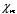. A current I flows down the inner conductor and returns along the outer one; in each case the current distributes itself uniformly over the surface (see Figure 1). Find the magnetic field in the region between the tubes. As a check, calculate the magnetization and bound currents and confirm that (together of course with the free currents) they generate the correct field.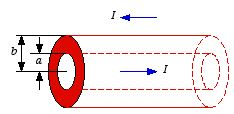Figure 1. Problem 1.

### Problem 2

a) A current I flows down a straight wire of radius R. If the wire is made of linear material (copper or aluminum) with susceptibility, and the current is distributed uniformly, what is the magnetic field a distance r from the center?
b) Find all the bound currents.
c) What is the net bound current flowing down the wire?

### Problem 3

Notice the following parallel: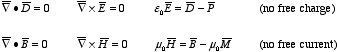Thus, the transcription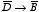,,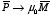, and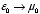turns an electrostatic problem into an analogous magnetostatic one. Use this observation, together with your knowledge of the electrostatic results, to calculate
a) The magnetic field inside a uniformly magnetized sphere.
b) The magnetic field inside a sphere of linear magnetic material in an otherwise uniform magnetic field.
c) The average magnetic field over a sphere, due to steady currents within the sphere.

### Problem 4

A familiar toy consists of donut-shaped permanent magnets (magnetization parallel to the axis), which slide frictionless on a vertical rod (see Figure 2). Treat the magnets as dipoles, with mass M and dipole moment.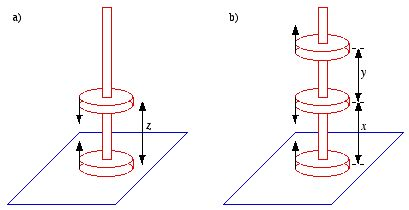Figure 2. Problem 4.
a) If you put two back-to-back magnets on the rod, the upper one will "float" - the magnetic force upward balancing the gravitational force downward. At what height z does it float?
b) If you now add a third magnet (parallel to the bottom one), what is the ratio of the two heights? (Determine the actual number, to three significant digits).

### Problem 5

At the interface between one linear magnetic material and another magnetic material the magnetic field lines bend (see Figure 3). Show that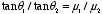, assuming there is no free current at the boundary.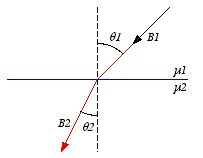Figure 3. Problem 5.